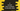# C program to convert hour to minute and seconds value## C program to convert hour to minute and seconds value:

In this post, we will learn how to convert a hour value to minute and seconds in C. It will take the hour value as the input, convert it to minute and seconds and print the result.

With this problem, you will learn how to take user inputs in C and how to do simple mathematical calculations in C.

### Algorithm:

We know that 1 hour is equal to 60 minutes and 1 minute is equal to 60 seconds. So, we can multiply the hour value by 60 to convert it to minute value and we can multiply it by 60 * 60 to convert it to seconds.

So, our program will take the hour value as input from the user and print the minute and seconds value.

### C program:

Let’s take a look at the final C program:

``````#include <stdio.h>

int main()
{
int hour, min, sec;

printf("Enter the hour value: ");
scanf("%d", &hour);

min = hour * 60;
sec = min * 60;

printf("Minutes : %d\n",min);
printf("Seconds : %d\n",sec);

return 0;
}``````

Here,

• We have initialized three integer values hour, min and sec to hold the hour, minute and second values.
• This program takes the hour value as input from the user. It asks the user to enter the value and by using scanf, it stores the value in the variable hour.
• It then converts the hour value to minute by multiplying it with 60.
• Similarly, it converts the minute value to seconds by multiplying it with 60. We can also multiply the hour value with 60 * 60 to get the seconds value.
• The last two printf statements are used to print the minute and second values we calculated before.

### Sample output:

If you run this program, it will print outputs as like below:

``````Enter the hour value: 10
Minutes : 600
Seconds : 36000``````

You can enter any hour value, it will convert that value to minutes and seconds and print the result.

I hope that you learned how to take user inputs and how to do simple mathematical calculations in C. You can use the same process to do any other type of conversions as well.